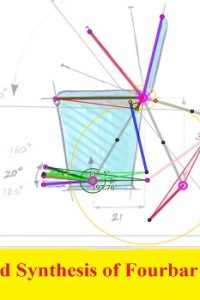اسم المؤلف
L. Roy, A. Sen, R. P. Chetia and M. J. Borah
التاريخ
14 سبتمبر 2021
المشاهدات
التقييم(لا توجد تقييمات)Loading...

بحث بعنوان
Analysis and Synthesis of Four Bar Mechanism
L. Roy, A. Sen, R. P. Chetia and M. J. Borah
Department of Mechanical Engineering,
National Institute of Technology
Silchar-788010, India
*Corresponding Author e-mail: [email protected]
Abstract
Computer programme and Genetic algorithm is used in analysis and
synthesis of a planar four-bar mechanism. Link dimensions were varied to
obtain coupler path maps. Position, velocity, acceleration and the force at the
coupler point at various input angles were obtained and the designed path is
utilized in the working models. Plot of variation of the link angles, variation
of velocity and acceleration of the coupler point with the crank angle is
obtained. It is found that ratio of velocity of crank and coupler point varies
between 4.48 and 0.38, acceleration of the coupler point is maximum. The
four bar mechanism can be used to transfer small jobs at regular interval of
time. With addition of another four bar mechanism with this, a papad making
machine is shown as an useful application.
Keywords: coupler curves, coupler point, Grashof’s law, four bar mechanism.
Notation
OAPBC the complete four bar mechanism
P coupler point
a crank
c rocker
e length of one of the sides of the ternary link
f length of the line joining A and C
θ angle between the a and d
β the coupler angle
γ angle made by the link b with the hz.line passes through the point A
RA,RP position vector of the point A, P
RPA the relative position of point P w.r.t point A
VA Velocity of point A
VP Velocity of point P
VPA Velocity of point P w.r.t point A
AA Acceleration of the point A
AP Acceleration of the point P
APA Acceleration of the point P w.r.t point A
1 Acceleration of the crank
2 Acceleration of the coupler
3 Acceleration of the rocker
φ angle made by the line joining point A and C with the horizontal line passes
through the point A.
Conclusion
1) The new maps developed can be used for synthesis of four bar mechanism .It is
easier to follow the synthesis procedure.
2) In comparison with the crank velocity, the velocity attained by the coupler point
is 4.48 times the crank velocity and the minimum velocity is 0.38 times the
crank velocity.
3) The acceleration of the coupler point varies between 51 cm/s2 and 3758 cm/s2 in
spite of zero acceleration of the crank
4) The magnitude of force at the coupler point varies between 4.11099N and
0.7797 N
5) Total distance traveled by the coupler point is 19.9 cm
6) The results obtained from the programme matches with the model prepared.
7) The design developed can be used for transporting small objects at regular
interval of time. It can be also be used or papad making machine .

كلمة سر فك الضغط : books-world.net